Knot theory

IV. Knot invariants: Classical theory

In this lesson, we define some classical knot invariants.

Section1. Minimum number of crossing points

A regular diagram of a knot K has at most a finite number of crossing points. However, this number c(D) is NOT a knot invariant. For example, the trivial knot has two regular diagrams D and E, which have a different number of crossing points, fig. 32.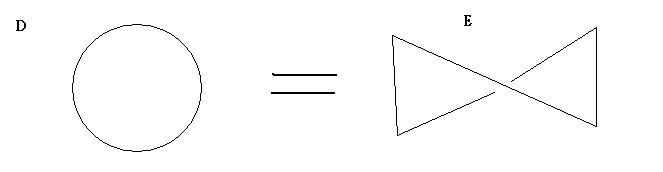fig. 32. the number of crossing points of D and E are respectively,  c(D)=0, but c(E)=1

Consider, instead, all the regular diagrams of a knot K, let c(K) be the minimum number of crossing points of ALL regular diagrams. This c(K) is a knot invariant. Mathematically speaking, we use the following equation to stand for the formula for such an expression, where S is the set of all regular diagrams of K :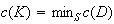Theorem 4.1    c(K) is a knot invariant.

Note: The above quantity is called the minimum number of crossing points of K. A regular diagram of K that has exactly c(K) crossing points is said to be the minimum regular diagram of K. For example, if K is a trivial knot, then c(K) = 0 .

Proof:    Suppose that D1 is the minimum regular diagram of K1. Let K2 be a knot that is equivalent to K1, and suppose that D2 is its minimum regular diagram. Since we can think of D2 as a regular diagram of K1 (since K1 and K2 are equivalent), from the definition we have that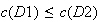However, since D2 is a regular diagram of K2, it again follows from the definition that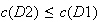Hence, combining these two inequalities, we obtain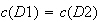That is, c(D1) is the minimum number of crossing points for all knots equivalent to K. Consequently, it is a knot invariant.

Exercise 4.1    Show that for c(D)=0, 1, 2, the trivial knot is the only knot that possesses a regular diagram D with one of these values.         ( Hint: It is pretty clear for c(D)=0. For the other two values, use elementary knot moves/Reidemeister moves to get back the trivial knot )

Exercise 4.2    Show that the trefoil knot, K, has c(K)=3. Further, show that among all knots, the trefoil knot is the only one with c(K) =3. (For the latter part, again, suppose you have such a knot with three crossing points, you should be able to deduce that trefoil knot is the only available choice, by using elementary knot moves. )

In general, there is no known method to determine c(K). It has only been computed for some specific types of knots. The difficult hinges on the fact that when the knot is very complicatedly knotted, then we are forced to deal with a gigantic number of regular diagrams which are equivalent to the original one. So the set S, in the definition of the minimum regular crossing points of knot, is just huge and extremely difficult to handle.

Section2. The bridge number

At each crossing point of a regular diagram D of a knot K (or link), let us remove from D a fairly small segment AB that passes over the crossing point. The result of removing these segments is a collection of disconnected (without any crossing points) polygonal curves, see fig. 33. We may think of the original regular diagram, D, as the resulting diagram that occurs when we attach the segments AB, that pass over to the endpoints of these disconnected polygonal curves on the plane.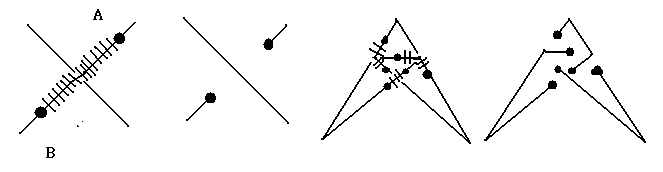fig. 33. The crossed lines in the first and the third pictures stand for the bridges, which pass over the diagram. To put it in the other way, we can say that the first and the third pictures are the regular diagrams of their respective original knots after attaching the crossed segments (which pass over) to the endpoints of the disconnected polygonal curves in the second and the fourth pictures, respectively.

Since these segments AB pass over the segments on the place, these segment AB are called bridges. For a given D, the number of bridges is called the bridge number. Mathematically, the bridge number is defined as br(D)

Example     The minimum bridge number of fig. 34 is 2. It is called the hopf link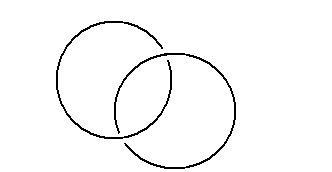fig. 34

Exercise 4.3

(A)    Show that     br(K1)=3,         br(K2)=2

(B)    By manipulations using your hands, show that K1 is equivalent to K2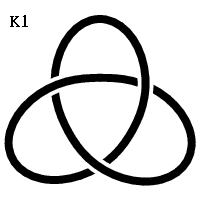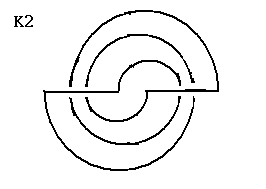fig. 35

By exercise 4.3, we know that bridge number is NOT a knot invariant. However, as in the previous section, if we consider all the regular diagrams for a given knot, then the minimum bridge number over all these regular diagrams of a given knot is a knot invariant. Mathematically, define the minimum bridge number by the following formula, where S is the set of all regular diagrams of a given knot K: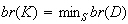Theorem 4.2    br(K) is a knot invariant.

Exercise 4.4    By considering the proof of theorem 4.1, prove theorem 4.2 by similar method.

Exercise 4.5    Show that if br(K)=1, then K is the trivial knot, and the trivial knot is the only knot with bridge number equal to 1

Exercise 4.6    Show that if L is a n-component link (that is, consisting of n knots) then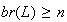In the specific case if br(K)=2, there are many knots with this bridge number, including the trefoil knot (look at the two representations in fig. 35 again, we knot that the minimum bridge number of the trefoil knot is at most 2. But by theorem 4.5, we know that the minimum bridge number must be 2) and the hopf link in fig.34. These knots, called for the obvious reasons 2-bridge knots, have been extensively studied, to the point that they have been completely classified. In general, however, no method has yet been found to allow us to determine the minimum bridge number for a random knot K. The reason is again, that the set S consisting of all regular diagrams of a knot, is huge when the knot is highly knotted in a complicatedly way.

To end this section, let us state the following theorem, which is proved in Schubert [Sc]. First, recall the meaning of a connected sum, K1*K2 of two knots K1, and K2  from lesson 2.

Theorem 4.3    Suppose K1, K2 are two arbitrary, knots (or links). Then we have the following formula: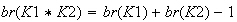Section3. The unknotting number

At one of the crossing points of a regular diagram, D, of a knot (or link) K exchange locally the over-crossing and under-crossing segments. Since this type of alteration is NOT an elementary knot move, in general what we obtain is a regular diagram of some other knots, see the following example, fig. 36.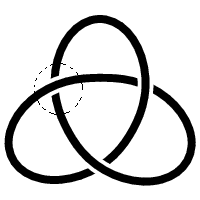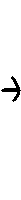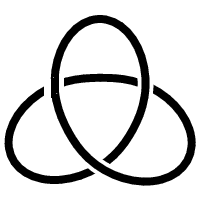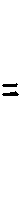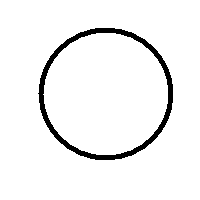fig. 36. If we exchange the under-crossing and the over-crossing segments within the small dotted circle in the first picture, the subsequent regular diagram can readily be seen as the trivial knot.

The following theorem is stated without a proof. For a proof, see K. Murasugi [Mu].

Theorem 4.4    We can change a regular diagram D, of an arbitrary knot (or link) to the regular diagram of the trivial knot (or link) by exchanging the over-crossing and the under-crossings segments at several crossing points of D. Notice that it may also be necessary to use Reidemeister moves.

We define the unknotting number of D as the minimum number of unknotting operations that are required to change D into the regular diagram of the trivial knot (or link). Mathematically, we denote it by u(D), as might be expected, it is NOT a knot invariant. For evidence why it is NOT a knot invariant, do the following exercise.

Exercise 4.7

(A)    By manipulations, show that the two knots in fig. 37 are equivalent.

(B)    Show that the first diagram has unknotting number 1, that is, it only requires 1 unknotting operation to change it into a trivial knot.

(C)    Show that the second diagram has unknotting number 2, that is, it requires 2 unknotting operations to change it into a trivial knot.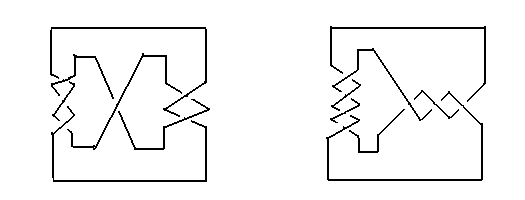fig. 37

As in the previous two sections in this lesson, if we consider all the regular diagrams for a given knot, then the minimum number of unknotting operations from all the regular diagrams is a knot invariant. Mathematically, we define minimum unknotting number as the minimum of unknotting numbers of all possible regular diagrams of a given knot K, recall that S is the set of all possible regular diagrams D for K: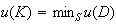Theorem 4.5    The minimum knotting number is a knot invariant.

Exercise 4.8    By considering the proof in theorem 4.1, prove theorem 4.5

If we exclude the trivial knot, then for all the other knots K, we have: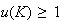However, to actually compute u(K) for a given knot is very difficult. Even for very specific types of knots, there is virtually no method available (so you can say that the computation of this minimum unknotting number is every more difficult than the minimum bridge number and the minimum number of crossing points.).  To finish this section, let's try the following exercises on your own.

Exercise 4.9    Show that the knot in fig. 38 has minimum unknotting number at most 3.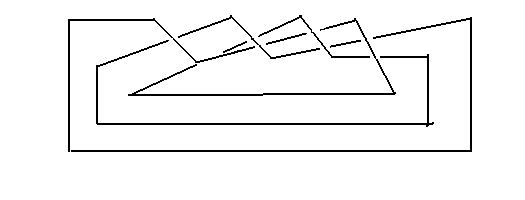fig. 38

Exercise 4.10    Show that the knot in fig. 39 has minimum unknotting number 1.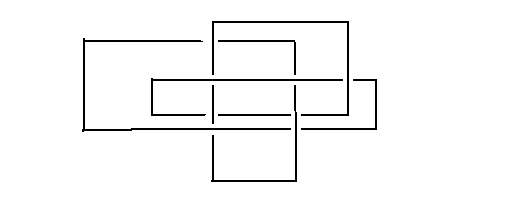fig. 39